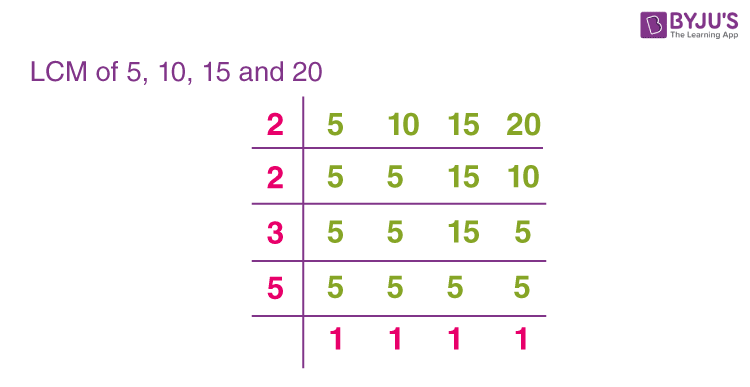# Find LCM of the 5, 10, 15, 20.

The Least Common Multiple(LCM) is a method to find the smallest common multiple between any two or more numbers. A common multiple is a number which is a multiple of two or more numbers.

## LCM of 5, 10, 15, and 20 by Common Division Method

• Step 1: Write the numbers 5, 10, 15, and 20 horizontally by separating them with a comma.
• Step 2: Choose the smallest prime number that divides at least any one of them.
• Step 3: Write the quotient in the next row just below the numbers. If any of the numbers are not divided, we will bring it down directly.
• Step 4: We will stop it when we don’t have a prime number to divide the given numbers.
• Step 5: The Least common multiple of 5, 10, 15, and 20 will be the product of primes by which we have divided.So, by common division method, we have LCM of 5, 10, 15, and 20 = 2 × 2 × 3 × 5 = 60(9)(2)